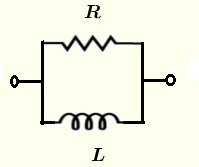# Parallel RL circuit Impedance Calculator

A calculator to calculate the equivalent impedance of a resistor and an inductor in parallel. The impedance is given as a complex number in standard form and polar forms.

  

## Formulae for Parallel R L Circuit Impedance Used in Calculator and their UnitsLet $f$ be the frequency, in Hertz.
The angular frequency is given by
$\omega = 2 \pi f$ , in rad/s
The inductive reactance
$X_L = \omega L$ , in ohms $(\Omega)$
The impedance of the inductor $L$ is given by
$Z_L = j \omega L$
Let $Z$ be the equivalent impedance to the parallel RL circuit shown above and write it in complex form as follows
$\dfrac{1}{Z} = \dfrac{1}{R} + \dfrac{1}{Z_L}$
$Z = \dfrac{R Z_L}{R + Z_L} = \dfrac{ j R \omega L }{R+j \omega L } = \dfrac{1}{\dfrac{1}{R} - j \dfrac{1}{\omega L}}$

The formulae for the modulus $|Z|$ and argument (or phase) $\theta$ of $Z$ are given by

Modulus: $|Z| = \dfrac{1}{\sqrt{ \dfrac{1}{r^2} + \dfrac{1}{\omega^2 L^2}}}$ in ohms $(\Omega)$

Argument (Phase): $\theta = \arctan \left( \dfrac{ R }{\omega L} \right)$ in radians or degrees

## Use of the calculator

Enter the resistance, the capacitance and the frequency as positive real numbers with the given units then press "calculate".

 Resistance R = 50 mΩ Ω KΩ MΩ Inductance L = 20 μH mH H Frequency f = 1.5 GHz MHz kH Hz mHz Number of Decimals 4